Tawnypelt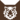虎族 (Q645)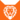狮族 (Q646)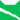雷族 (Q627)[临时][避难] [避难]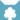天族 (Q632)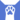反抗者 (Q4414)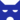影族 学徒影族 武士影族 猫后雷族 武士[临时]影族 武士影族 副族长
（继任自虎星 (Q2687)天族 武士影族 副族长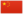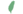黄崽 Tawnykit  小褐
 黄爪 Tawnypaw  褐掌
 褐皮 Tawnypelt  褐皮

Q2647：影族母猫，原雷族猫，自《疑云重重》登场的角色

## 细节

### 外貌

• 她是一只身形精瘦、:3毛色斑驳、:16玳瑁色:猫物表和白色:82相间的母猫，:12有着清澈:309的绿色眼睛。:53

### 作者声明

• 维琪证实花楸掌之所以被选为褐皮的伴侣，是因为维琪为总是弄错他的性别感到抱歉。
• 凯特视她为暮棕色母猫。[请求来源]
• WarriorCats.com选出了二十四只猫进行2021年的绒毛娃娃投票，她排名第十八。

### 勘误

• 在幼崽时期，她被描述为如同母亲的浅姜色，:225但后来被更改为玳瑁色。维琪认为她应该是黄褐色或浅姜色。
• 她曾被错误地描述为虎斑、:130黄褐色、:3浅蕨色、:174浅色:184和浅棕色。:9章
• 她曾被错误地描述为琥珀色眼睛。:152-153:271
• 她曾在成为武士后:38被错误地以学徒名称呼。:3:178-179

## 登场书目

• 疑云重重 (Q60)[配角][首次登场🐱]
• 风起云涌 (Q65)[配角]
• 险路惊魂 (Q66)[配角]
• 力挽狂澜 (Q67)[配角]
• 午夜追踪 (Q139)[主要角色]
• 新月危机 (Q140)[主要角色]
• 重现家园 (Q141)[配角]
• 星光指路 (Q142)[配角]
• 黄昏战争 (Q143)[配角]
• 日落和平 (Q144)[配角]
• 预视力量 (Q152)[猫物闲角]
• 暗河汹涌 (Q153)[对话提及]
• 驱逐之战 (Q154)[配角]
• 天蚀遮月 (Q155)[配角]
• 暗夜长影 (Q156)[配角]
• 拂晓之光 (Q157)[配角]
• 第四学徒 (Q158)[配角]
• 战声渐近 (Q159)[配角]
• 暗夜密语 (Q160)[配角]
• 月光印记 (Q161)[猫物闲角]
• 武士归来 (Q162)[配角]
• 群星之战 (Q163)[配角]
• 学徒探索 (Q170)[对话提及]
• 雷影交加 (Q171)[配角]
• 天空破碎 (Q172)[配角]
• 极夜无光 (Q173)[配角]
• 烈焰焚河 (Q174)[配角]
• 风暴来袭 (Q175)[配角]
• 迷失群星 (Q3563)[配角]
• 静默冰融 (Q3579)[配角]
• 叠影重障 (Q3624)[配角]
• 暗由心生 (Q4183)[配角]
• 无星之地 (Q4385)[配角]
• 迷雾明光 (Q4621)[配角]
• 河翻浪涌 (Q4643)[配角]
• 天穹将倾 (Q4799)[配角]
• 火星的探索 (Q176)[配角]
• 黑莓星的风暴 (Q182)[配角]
• 虎心的阴影 (Q185)[配角]
• 鸦羽的拷问 (Q186)[猫物闲角]
• 松鼠飞的希冀 (Q3561)[猫物闲角]
• 灰条的誓言 (Q4057)[猫物闲角]
• 一星的告解 (Q4826)[配角]
• 冬青叶的故事 (Q187)[猫物闲角]
• 雾星的征兆 (Q188)[猫物闲角]
• 虎掌的愤怒 (Q190)[配角]
• 叶池的祈愿 (Q191)[猫物闲角]
• 鸽翅的沉默 (Q192)[猫物闲角]
• 褐皮的族群 (Q3566)[主人公][主要角色][视角人物]
• 蛾翅的秘密 (Q3628)[配角]
• 黛西的至亲 (Q3629)[猫物闲角]
• 斑毛的叛变 (Q4337)[猫物闲角]
• 黑脚的清算 (Q4336)[配角]
• 河族阴云 (Q4311)[仅于回忆]
• 变革之风 (Q4411)[配角]
• 族群的秘密 (Q199)[配角]
• 族群的猫 (Q200)[配角]
• 族群的战争 (Q202)[配角]
• 终极指南 (Q204)[配角]
• 族群决定 (Q4580)[配角]

更多资料：褐皮/周边

## 外部链接

### 官方文章

• 哪名武士最值得？ （投票胜者）
• 选个绒毛娃娃 （投票选项）
• 向猫妈妈致敬 （提及）
• 未成立的守则 （隐射）
• 消失的传统 （隐射）
• 新猫页面－褐皮 （专题）
• 团结则存，分裂则亡 （隐射）
• 共同的纽带 （提及）
• 族群还是至亲？ （提及）
• 预料之外的剧情 （隐射）
• 你至亲的至亲 （提及）
• 当征兆为假 （提及）
• 我们的边界之外 （隐射）
• 解读征兆 （隐射）
• 选个大型绒毛娃娃：续集 （投票选项）
• 只是故事而已 （隐射）
• 沉重的要求 （提及）
• 急水部落 （提及）
• 大千世界 （隐射）
• 跨越边界 （隐射）
• 浪迹天涯 （隐射）
• 《新预言》主要角色盘点 （专题）
• 揭开猫武士大本营的面纱 （提及）
• 追忆往昔 （提及）
• 血缘之外 （提及）
• 真正的武士？ （专题）
• 选个绒毛娃娃2021 （投票选项）
• 介绍你们选出的2021绒毛娃娃 （提及）
• 我们所铭记的死亡 （隐射）
• 平衡光明的黑暗 （提及）
• 武士的誓词 （隐射）
• The Forest Map: WindClan （隐射）
• 换族者：他们会留下吗？ （提及）
• 亮心与《雷族神偷》 （提及）
• ## 参考文献

这篇文章基于CC BY-SA 3.0许可使用了猫武士维基（英语）Tawnypelt一文中的部分内容。
1. 《疑云重重》第二十二章 (Q821)
2. 《烈火焚河》第十章
3. 《险路惊魂》第二十章
4. 返回族群 (Q212)
5. 鸽翅的沉默 (Q192)学徒探索 (Q170)
6. 极夜无光 (Q173)风暴来袭 (Q175)
7. 风暴来袭 (Q175)松鼠飞的希冀 (Q3561)
8. 午夜追踪
9. 雷影交加
10. 终极指南
11. 天蚀遮月
12. 迷失群星
13. 艾琳·亨特访谈3
14. 介绍你们选出的2021绒毛娃娃〉(2021-05-20). 站内截图. WarriorCats.com. 原页面
15. Su Susann (2016-09-30). 站内截图. Facebook . 原页面 归档于 2017-10-31. “As I said, Adderfang is a descandant of Spottedpelt and Pinestar is, trough the blood of his father, related to Gorseclaw.”
16. 烈焰焚河
17. 风暴来袭
18. 第四学徒
19. 黑莓星的风暴
20. 学徒探索
21. 虎心的阴影
22. 重现家园
23. 驱逐之战
24. 褐皮的族群
25. Official Website (2023-11-17). Tawnypelt . WarriorCats.com .
26. 疑云重重
27. Vicky Holmes: 1/3 of the Erin Hunters! (2013-08-21). Tessa Roberts - Why, both with the name Tawnypelt and described as... . 访问于 2016-10-06. “Tawnypelt should be tawny/pale ginger throughout.”
28. 险路惊魂
29. 黄昏战争
30. 虎掌的愤怒
31. 力挽狂澜
32. 新月危机
33. 火星的探索